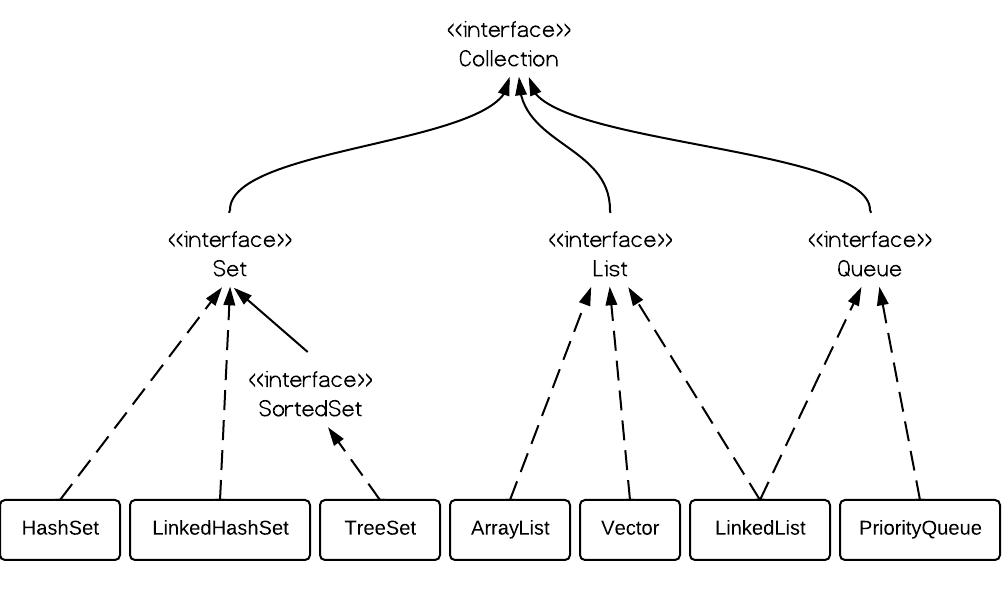# HashSet vs. TreeSet vs. LinkedHashSet

· Java Zone · Interview
Save
148.34K Views

in a set, there are no duplicate elements. that is one of the major reasons to use a set. there are 3 commonly used implementations of set in java: hashset, treeset and linkedhashset. when and which to use is an important question. in brief, if we want a fast set, we should use hashset; if we need a sorted set, then treeset should be used; if we want a set that can be read by following its insertion order, linkedhashset should be used.

### 1. set interface

set interface extends collection interface. in a set, no duplicates are allowed. every element in a set must be unique. we can simply add elements to a set, and finally we will get a set of elements with duplicates removed automatically.### 2. hashset vs. treeset vs. linkedhashset

hashset is implemented using a hash table. elements are not ordered. the add, remove, and contains methods has constant time complexity o(1). treeset is implemented using a tree structure(red-black tree in algorithm book). the elements in a set are sorted, but the add, remove, and contains methods has  time complexity of o(log (n)). it offers several methods to deal with the ordered set like first(), last(), headset(), tailset(), etc.  linkedhashset is between hashset and treeset. it is implemented as a hash table with a linked list running through it, so it provides the order of insertion. the time complexity of basic methods is o(1).

### 3. treeset example

``````treeset tree = new treeset();

iterator iterator = tree.iterator();
system.out.print("tree set data: ");
while (iterator.hasnext()) {
system.out.print(iterator.next() + " ");
}
``````
output is sorted as follows:
`` tree set data: 12 34 45 63  ``
now let's define a dog class as follows:
``````class dog {
int size;

public dog(int s) {
size = s;
}

public string tostring() {
return size + "";
}
}
``````
let's add some dogs to treeset like the following:
``````import java.util.iterator;
import java.util.treeset;

public class testtreeset {
public static void main(string[] args) {
treeset dset = new treeset();

iterator iterator = dset.iterator();
while (iterator.hasnext()) {
system.out.print(iterator.next() + " ");
}
}
}
``````
compile ok, but run-time error occurs:
`````` exception in thread "main" java.lang.classcastexception:
collection.dog cannot be cast to java.lang.comparable
at java.util.treemap.put(unknown source)
at collection.testtreeset.main(testtreeset.java:22) ``````

because treeset is sorted, the dog object need to implement java.lang.comparable's compareto() method like the following:
``````class dog implements comparable{
int size;

public dog(int s) {
size = s;
}

public string tostring() {
return size + "";
}

@override
public int compareto(dog o) {
return size - o.size;
}
}
``````
the output is:
``1 2 3  ``

### 4. hashset example

``````hashset dset = new hashset();
iterator iterator = dset.iterator();
while (iterator.hasnext()) {
system.out.print(iterator.next() + " ");
}
``````
output:
``5 3 2 1 4  ``

note the order is not certain.

``````linkedhashset dset = new linkedhashset();
iterator iterator = dset.iterator();
while (iterator.hasnext()) {
system.out.print(iterator.next() + " ");
}
``````
the order of the output is certain and it is the insertion order.
``2 1 3 5 4  ``

### 6. performance testing

the following method tests the performance of the three class on add() method.
``````public static void main(string[] args) {
random r = new random();

hashset hashset = new hashset();
treeset treeset = new treeset();

// start time
long starttime = system.nanotime();
for (int i = 0; i < 1000; i++) {
int x = r.nextint(1000 - 10) + 10;
}
// end time
long endtime = system.nanotime();
long duration = endtime - starttime;
system.out.println("hashset: " + duration);

// start time
starttime = system.nanotime();
for (int i = 0; i < 1000; i++) {
int x = r.nextint(1000 - 10) + 10;
}
// end time
endtime = system.nanotime();
duration = endtime - starttime;
system.out.println("treeset: " + duration);

// start time
starttime = system.nanotime();
for (int i = 0; i < 1000; i++) {
int x = r.nextint(1000 - 10) + 10;
}
// end time
endtime = system.nanotime();
duration = endtime - starttime;

}``````

from the output below, we can clearly wee that hashset is the fastest one.
``````hashset: 2244768
treeset: 3549314